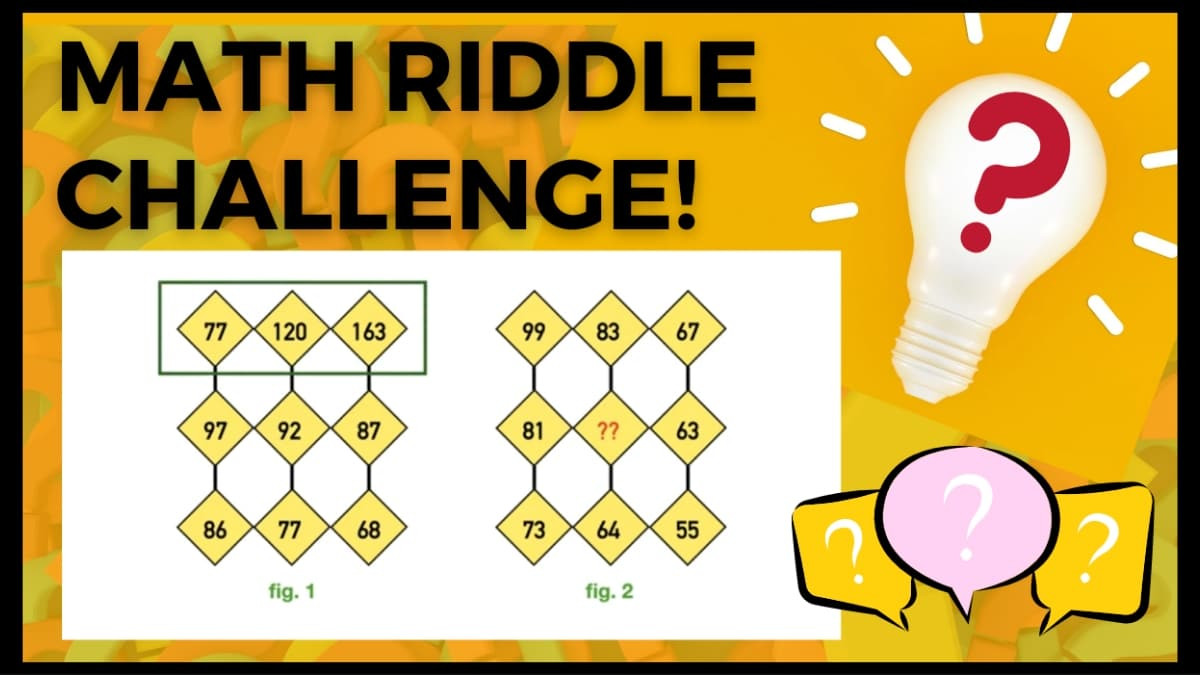# Math Riddles: Do You Have A High IQ? Solve These Figures and Find the Missing Number!

Math Riddles Challenge: Can you find the missing numbers in the place of the question mark in this math puzzle figure? Check Your IQ!Math Riddles: Do You Have A High IQ? Solve These Figures and find the missing number!

Math Riddles Challenge: Can you find the missing numbers in the place of the question mark in this math puzzle figure? Check Your IQ! Cryptic Math Riddles are the best to train your brain for all math lovers. Well, we bring you another set of tricky math puzzles where one has to find the missing numbers in the place of the question marks.

Can you do it? Let us see if you can find the missing number in 30 seconds!

### What will be at the place of ? Mark. Suppose that Fig.2 works in the same pattern as Fig 1.

Here there are two figures!

In each figure, numbers are represented in a certain pattern and you are supposed to find the number at the place of the question mark in figure 2.

You have 30 seconds to solve this cryptic equation. Give it a try!

3…

2…

1…

### Let's look at the solution now!

It is important to understand the right approach to attempt this kind of problem. As you can notice here there are series of numbers represented horizontally and vertically. If you observe carefully, you may think there is no clue about the numbers represented vertically but eventually, you’ll notice that there is a clear pattern observed in the numbers represented horizontally!

## Let's observe Figure 1

Fig 1

If we see the first row of numbers in Figure 1 you can apply the logic of adding the first and last numbers in the blocks and then dividing the result by two. You will see that the resulting number is the second number in the row.

77 + 163= 240

Now you can divide the result by 2

240÷2 = 120

The resulting number is the second number which is 120.

Similarly in the second row of numbers at first and last number is 97 plus 87 which is equal to 184 divide the result by 2 which is 184 over 2 resulting number is the second number which is 92

Solution:

97+87=184

184÷2 = 92

## Let's Observe Figure 2

Let's look at the second figure with the mystery number!

Fig. 2

Similarly in figure 2 numbers are following the same pattern as in figure 1. To get the missing number you can apply a similar logic.

To confirm that both figures adhere to the same logic let's look at the first row of the second figure.

When we add the first and the last numbers in the blocks made in the first row we can see that it applies the same logic.

If you add 99 and 87 and then divide the result by 2 you will get the middle number 83

99+87= 166

166÷2= 83

Hence, proved

Now consider the row with the missing number that is the second row

Add the first and last number which is 81 plus 63 which is equal to 144 now divide it by 2 which results in 72 which is the missing number year thus the answer to this puzzle is 72.

Solution:

81+63=144

144÷2=72

### Missing Number: 72

GREAT WORK!

ALSO CHECK OUT: Math riddles with answers: Math is tough, but fun too! Let these math riddles prove to you how fun math can be!

Brain Teasers: How good were you at geography? Let’s find out today!

Math riddles with answers: These math riddles are a must-try!

Get the latest General Knowledge and Current Affairs from all over India and world for all competitive exams.
खेलें हर किस्म के रोमांच से भरपूर गेम्स सिर्फ़ जागरण प्ले पर